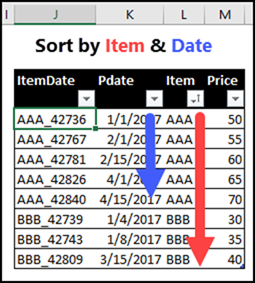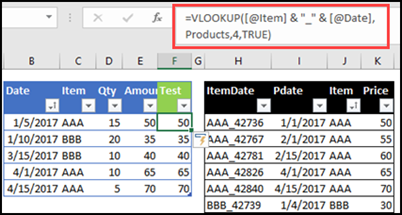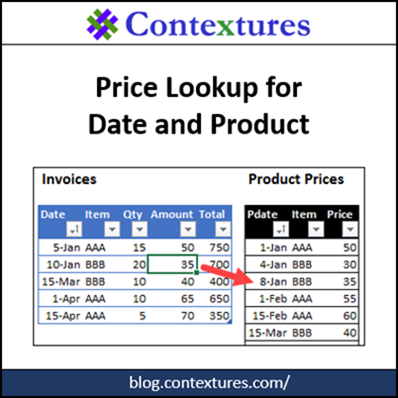# Excel Price Lookup Date Product Name

If there's just one price per product in an Excel lookup table, you could use the INDEX and MATCH functions to to get that price. But what if the price changes occasionally, and your pricing list has multiple dates and prices for each product? How can you do a product price lookup based on invoice date and product name?

### Find the Latest Price

Someone asked that question on my Contextures blog last week, on my post that shows how to find the latest price for a specific product in Excel.

Before we tackle the new problem, here is the example from that old post, where we wanted the LATEST price. The screen shot below shows a lookup table, with product prices and dates.In that example, I used two formulas:

1. MAX and IF (array-entered with Ctrl+Shift+Enter) to find the latest date for a product
=MAX(IF(\$A\$2:\$A\$9=A12,\$B\$2:\$B\$9))
2. Then, SUMIFS to find the product's price on that date.
=SUMIFS(\$C\$2:\$C\$9,\$A\$2:\$A\$9,A12,\$B\$2:\$B\$9,B12)

Here's the result of a lookup for Pens, in that example. The formula ignores all the earlier prices for Pens, and returns the latest one.### Find the Matching Date for an Invoice

The new question had a different twist – there was an on-going list of invoices, and it needed a lookup formula to find the product price based on the invoice date.

In the screen shot below, the invoice list is at the left, and the product pricing lookup is on the right.

• For the "BBB" sale on Jan. 10th, we need the Jan 8th price from the lookup table.
• The Mar 15h sale of BBB needs the Mar 15th price.### How Would You Solve It?

In my Excel newsletter this week, I posted my solution, and asked if anyone had another way to get the correct prices.

My formula was a long, complicated, array-entered monstrosity. I figured someone could find a simpler solution. Brace yourself – here it is:

=INDEX(Products[Price], SMALL(IF(Products[Item]=[@Item], IF(Products[Pdate]<=[@Date], ROW(Products[Pdate]) - ROW(Products[[#Headers],[Pdate]]))), COUNTIFS(Products[Item],[@Item],Products[Pdate], "<="&[@Date])))

It works, but it's very difficult to read and understand. Also, array formulas break easily, if someone presses Enter, instead of Ctrl+Shift+Enter

### Alternative 1 – VLOOKUP

Fortunately, some smart and creative people read my newsletter, and they sent me their solutions.

Van V and Tim O both suggested using VLOOKUP. You'll need to add a column at the left of the pricing table, with a formula to combine the product name and date.

It's not necessary, but I added an underscore between the name and date, as a separator.

= [@Item] & "_" & [@Pdate]

Next, sort the price list by product (item) and date – this is crucial to making this method work.Then, in the Invoice list, use a VLOOKUP formula with an approximate match, to get the correct price.

• =VLOOKUP([@Item] & "_" & [@Date], Products,4,TRUE)

I left my original formula in column E, for comparison, and add the VLOOKUP in column F.

The VLOOKUP worked perfectly, so it's a good option, if:

• you can add a column to the pricing table,
• and remember to sort it by Item and Date.

Thanks to Van and Tim for sending their VLOOKUP solutions.### Alternative 2 – INDEX/MATCH

Paul B and Tim O sent their solutions too, and they both used INDEX and MATCH. The setup is similar to the VLOOKUP solution:

• add a column to the pricing table, to combine the item and date. However, it doesn't need to be on the left – it can be anywhere in the table.
• pricing table must be sorted by item name and date (Tim suggested a macro to do that, so it's easier).

Here is the formula for the invoice table, to pull the correct price from the pricing table:

• =INDEX(ProductsLU[Price], MATCH([@Item] &[@Date], Products[ItemDate],1))

### All-In-One Formulas

Thanks to David P and Leonid K, who also sent formulas, and these didn't require any changes to the pricing table. All three of their formulas are better than the one that I created – shorter and easier to read.

1) David's first formula uses LOOKUP:

• =LOOKUP(1,1/FREQUENCY(0,1/(1+(Products[Item]=[@Item])*(Products[Pdate]<=[@Date])*Products[Pdate])),Products[Price])

2) David's second formula uses ROUND, and is array-entered (Ctrl++Shift+Enter):

• =ROUND(MOD(MAX( IF( (Products[Item]=[@Item])* (Products[Pdate]<=[@Date]), Products[Pdate] + Products[Price]/1000000)), 1)* 1000000,5)

3) Leonid's formula is an INDEX/MATCH formula:

• =INDEX(Products[Price],MATCH(1,1/((Invoice[@Item]=Products[Item])*(Products[Pdate]<=Invoice[@Date])*Products[Pdate])))

### Which Solution Would You Choose?

With so many formula options, which one would you choose for your workbook?

• The VLOOKUP and first INDEX/MATCH formulas are easiest to understand. However, they require changes and maintenance to the pricing table.
• The All-In-One formulas are a bit more complicated, but don't require any changes to the pricing table
• My original formula works, but it's the longest, and most complicated. I'm fond of it though, after all the deep thought that went into it!

### The Original Formula

A couple of people asked how my original formula works, so here is a description of the key pieces.

• The INDEX function will return a specific item in an array (range of cells), and the SMALL function tells it which item to return (row number in the range).
• The SMALL function returns the nth smallest number in an array , and COUNTIFS calculates that “n”.
• The ROW function returns the worksheet row number for each matching item. To get the row within the pricing list, we subtract the row number for the pricing list header.
• To see the row numbers that the SMALL function can choose from:
• In cell E6, select the “array” part of the SMALL function in the formula bar, and press F9
• You will see this result: SMALL({FALSE;2;3;FALSE;FALSE;6;FALSE;FALSE}
• For items that match the criteria, the numbers show their positions in the price lookup table. Items that don’t match show as FALSE.
• The COUNTIFS calculates how many prices in the table have the same item, and a price date on or before the invoice date. NOTE: For this to work, the pricing table must be sorted by date
• To see the COUNTIFS result:
• With cell E6 still selected, in the formula bar, select the COUNTIFS part of the formula, and press F9
• The result is 3
• =INDEX(Products[Price],SMALL({FALSE;2;3;FALSE;FALSE;6;FALSE;FALSE},3))
• The 3rd smallest number in the array is 6, so the price from the 6th row is returned by the INDEX function.

### Get the Product Price Lookup Workbook

To get the workbook, with my original solution, and the better alternatives, go to the Sample Excel Files page on my Contextures website.

In the Functions section, look for FN0049 - Product Price Based on Date. The zipped file is in xlsx format, and does not contain any macros._________________

This site uses Akismet to reduce spam. Learn how your comment data is processed.